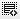## Quiz Question of L 440
Assume that a project's expected return is \$180 million in 5 years for an initial investment of \$100 million? How much debt should this project take to get a 10% ROI after debt payback? Assume 10% interest on the debt.

## 2 Answer(s)0

Since this is a quiz question, I will try my best to clarify the question withough giving away the answer -
Project needs \$100 mn investment
This \$100mn can be a combination of equity + debt
Obviously higher the debt, higher the ROI if the project is successful
Whatever debt you take has to pay back an annual interest of 10%
So you have your initial investment = \$100mn (subtract the debt from this to get the equity investment)
Then from you final returns, subtract the debt to paid back along with the interest expense.
So now you have your initial equity investment and final equity returns. and expected ROI. You should be able to backtrack the formula to find the right amount of debt.

Hope this is helpful0

Sir, but according to the question, the options given for the answer are incorrect. The answer calculated by taking the values given in the question does not match any of the answers given in the options.....

## Your Answer

Click on thisicon to add code snippet.

Email
Password